# Concave Lens

The lens is one of the significant discoveries made by humans. Although one can’t say when or how the lens was discovered, it is clear that ancient people realized that they could manipulate light using a piece of glass at some point in the past. For example, humans used a lens to make distant objects appear nearer; small objects appear larger and blurry objects appear clear (i.e. magnifying glasses and corrective lenses). Lenses used to accomplish these tasks fall into two categories of simple lenses as Convex and Concave Lenses. In this article, we will be closely looking into the Concave lens and its characteristics.

## What is a Concave Lens?

A concave lens is a lens that diverges a straight light beam from the source to a diminished, upright, virtual image. It can form both real and virtual images. Concave lenses have at least one surface curved inside. A concave lens is also known as a diverging lens because it is shaped round inwards at the centre and bulges outwards through the edges, making the light diverge. They are used to treat myopia as they make faraway objects look smaller than they are.

Watch the video below to learn how and why spectacles/contact lenses are used to correct vision defects and how exactly they affect the human eye. The demonstration in this video uses lasers and lenses to simulate what happens inside the human eye. For example, you will see how an eye with myopia (shortsightedness, nearsightedness) is different from a normal eye in the video. The demonstration also shows why a diverging lens is used to correct shortsightedness.

## Watch the video and learn about the working of spectacles### Concave Lens Formula

The lens formula is used to identify the nature and the position of the image formed by the concave lens. The lens formula is expressed as follows:

$$\begin{array}{l}\frac{1}{f}=\frac{1}{v}+\frac{1}{u}\end{array}$$

where f is the focal length, v is the distance of the image from the centre and u is the distance of the object from the centre.

Similarly, the magnification of the image is given by the equation,

$$\begin{array}{l}M=\frac{h_{i}}{h_{o}}=\frac{v}{u}\end{array}$$

where M is the magnification, hi is the height of the image and ho is the height of the object.

## Image Formation in Concave Lens

An object placed at infinity forms a virtual image at the focus. The size of the image is much smaller than that of the object.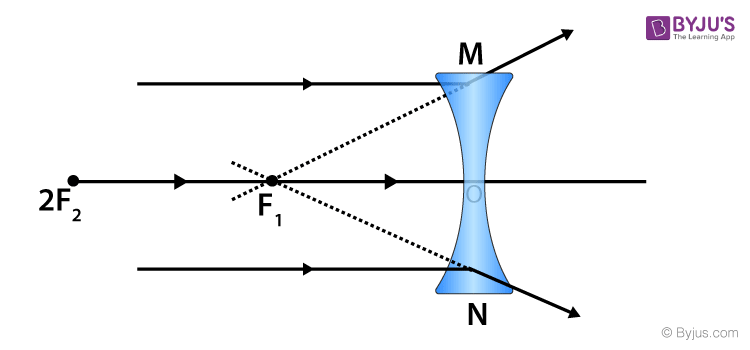An object placed at a finite distance from the lens forms a virtual image between the pole and the focus of the convex lens. As a result, the size of the image is smaller than that of the object.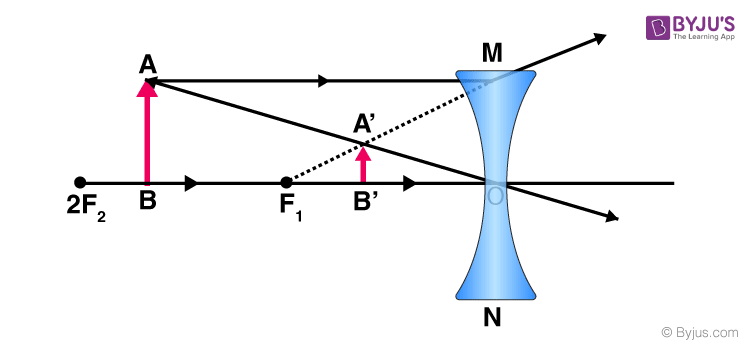## Watch the video and understand the concepts of Lens Formula, Magnification and Power of Lens## Uses of Concave Lens

Some uses of the concave lens are listed below:

### Used in Telescope

Concave lenses are used in telescopes and binoculars to magnify objects. As a convex lens creates blurs and distortion, telescope and binocular manufacturers install concave lenses before or in the eyepiece so that a person can focus more clearly.

### Used in Eye Glasses

Concave lenses are most commonly used to correct myopia which is also called nearsightedness. The eyeball of a person suffering from myopia is too long, and the images of faraway objects fall short of the retina. Therefore, concave lenses are used in glasses which correct the shortfall by spreading out the light rays before it reaches the eyeball. This enables the person to see far away objects more clearly.

### Used in Peepholes

Peepholes or door viewers are security devices that give a panoramic view if objects outside walls or doors. A concave lens is used to minimize the proportions of the objects and gives a wider view of the object or area.

To know more uses of a concave lens, click Concave Lens Uses.

 Similar articles that you might be interested in:

## Watch the video and revise all the important concepts in the chapter Light Reflection and Refraction Class 10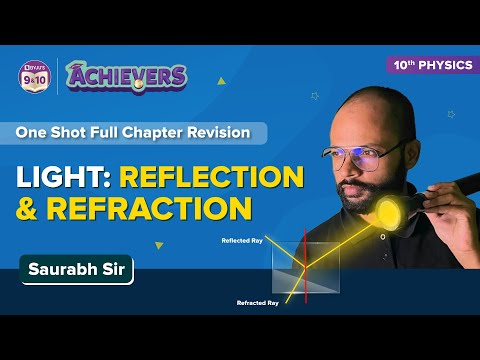## Frequently Asked Questions – FAQs

Q1

### What is Concave Lens?

A concave lens is a lens that diverges a straight light beam from the source to a diminished, upright, virtual image.
Q2

### How do Concave Lens correct Myopia?

A concave lens causes light rays to diverge before they strike the lens of the eye so that the image is formed on the retina.A concave lens is a lens that diverges a straight light beam from the source to a diminished, upright, virtual image.
Q3

### Where are Concave Lens used?

A concave lens is commonly used in telescopes, peephole and eyeglasses.
Q4

### Is Concave Lens converging or diverging?

A concave lens is diverging.
Q5

### What is the difference between concave and convex lens?

A convex lens is thicker at the centre and thinner at the edges. A concave lens is thicker at the edges and thinner at the centre.

## Recommended Videos

#### Previous year questions in the chapter Light Reflection and Refraction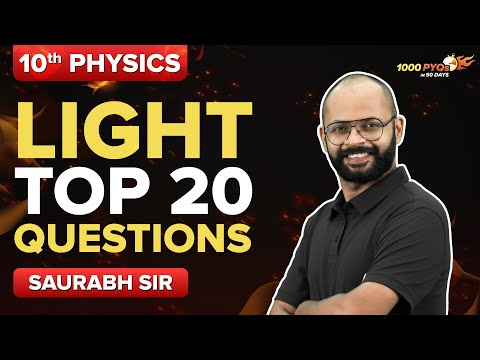#### Theory and important questions of the chapter Light: Reflection and Refraction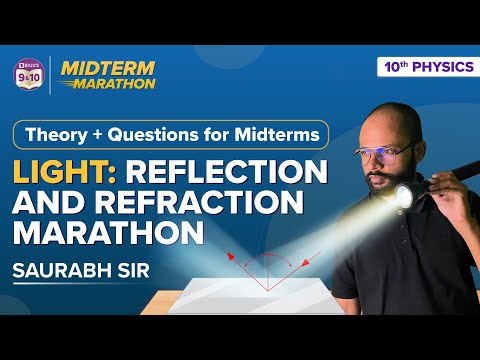Stay tuned to BYJU’S and Fall in Love with Learning!

Test Your Knowledge On Concave Lens!